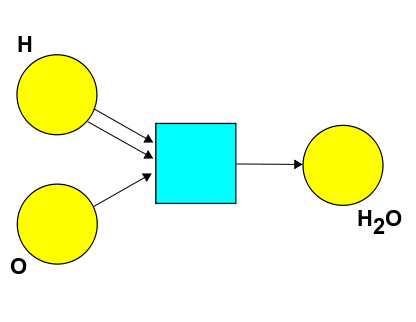Let me see if I get what you're saying about the difference between a Petri net and a wiring diagram!

Let's stick with the example where our resources are

* \$$H\$$
* \$$O\$$
* \$$H_2O\$$

The Petri netmakes \$$\mathbb N[S]\$$ into a commutative monoidal preorder by specifying the relation \$(a + 2n)[H] + (b + n)[O] + c [H_2O] \leq a [H] + b[O] + (c + n)[H_2O] \$ for all \$$a,b,c,n \in \mathbb N\$$.

The wiring diagram with 2 hydrogen wires and 1 oxygen wires going into a box and 1 \$$H_2O\$$ wire exiting the box would be a proof of the inequality \$2[H] + [O] \leq [H_2O].\$ Two of these wiring diagrams set up in parallel would be a proof of the inequality \$4[H] + 2[O] \leq 2 [H_2O].\$ And the wiring diagram with 2 hydrogen wires and 1 oxygen wires going into a box, 1 \$$H_2O\$$ wire exiting the box, and 1 oxygen wire running uninterrupted in parallel would be a proof of the inequality \$2[H] + 2[O] \leq [O] + [H_2O]\$